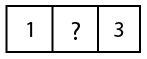Question 31

# Find the missing digit if it has 11 and 13 as factors ?Solution

In question given that the missing Number Has 11 and 13 as factor , so the number must be divisible by 11 and 13

By multiplying 11 and 13 we get 143, 143 is the only number that can have factor 11 and 13 ( having 3 at unit place and 1 at hundred place )